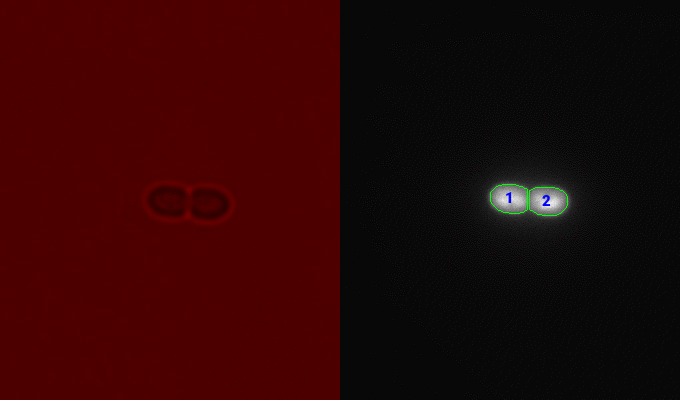# Linear Assignment Tracking Toolbox

## The problem

Cells move and divide as they grow under the microscope. This makes identifying and following an individual cell difficult.

## The solutionExample of cell tracking algorithm on a movie of growing cyanobacteria. Each cell on the right image shows a blue number which uniquely identifies the cell. Upon division, daughter cells are assigned new numbers.

To track an individual cell, I created a MATLAB toolbox which implements a tracking algorithm based on the Linear Assignment approach (Jaqaman et al. Nature Methods, 2008). Two classes are defined here: `LAPLinker` implements the object tracking algorithm and `TrackArray` implements a data structure to hold the resulting time-series data.

While this code is based on the original paper, several significant changes have been made:

• To solve the assignment problem, we used the Jonker-Volgenant algorithm (MATLAB implementation by Yi Cao). This algorithm allowed solutions to be found up to 10x faster compared to the Mukres algorihtm which was used in the original work.
• This code does not implement the full tracking algorithm in the original. Specifically, I omitted the gap closing, merging, and splitting step for simplicity.
• The code implements several custom metrics to identify cells, including using an overlap score, which is calculated based on the number of pixels in object masks. This approach worked much better than distance for cells which are non-motile.
• This code also identifies cell division using the same overlap score.

## Repository

Code, compiled MATLAB toolboxes, and instructions can be found here.

This toolbox has been used in a number of publications, including